Most Affordable JEE | NEET | 8,9,10 Preparation by Kota's Top IITian Doctor Faculties

# NCERT Solutions for Class 12 Physics Chapter 6 Electromagnetic Induction PDF - eSaral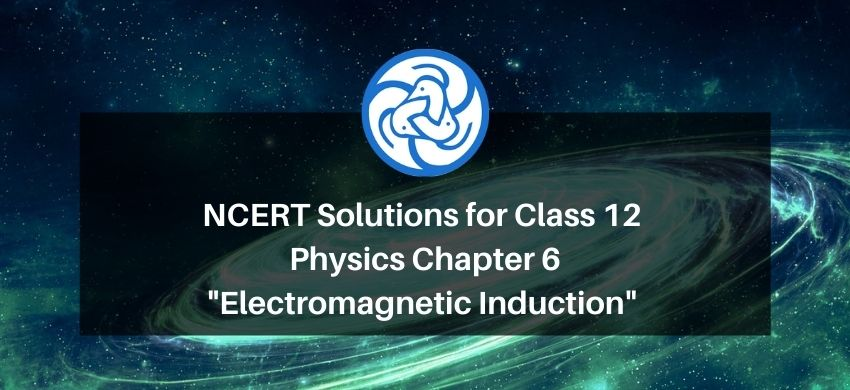`

# NCERT Solutions for Class 12 Physics Chapter 6 Electromagnetic Induction PDF

Hey, are you a class 12 student and looking for ways to download NCERT Solutions for Class 12 Physics Chapter 6 Electromagnetic Induction PDF? If yes. Then read this post till the end.

In this article, we have listed NCERT Solutions for Class 12 Physics Chapter 6 Electromagnetic Induction in PDF that are prepared by Kota’s top IITian Faculties by keeping Simplicity in mind.

If you want to learn and understand class 12 Physics Chapter 6 "Electromagnetic Induction" in an easy way then you can use these solutions PDF.

NCERT Solutions helps students to Practice important concepts of subjects easily. Class 12 Physics solutions provide detailed explanations of all the NCERT questions that students can use to clear their doubts instantly.

If you want to score high in your class 12 Physics Exam then it is very important for you to have a good knowledge of all the important topics, so to learn and practice those topics you can use eSaral NCERT Solutions.

So, without wasting more time Let’s start.

### Download NCERT Solutions for Class 12 Physics Chapter 6 Electromagnetic Induction PDF

Question 1. Predict the direction of induced current in the situations described by the following Figs. $6.18(\mathrm{a})$ to (f).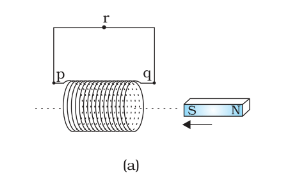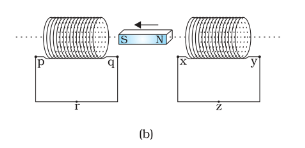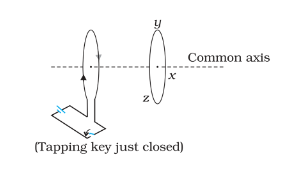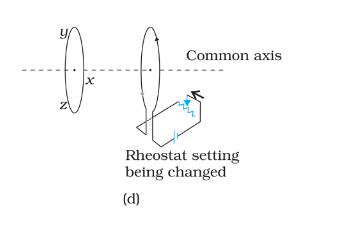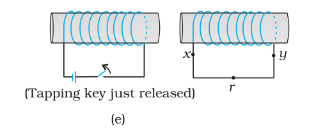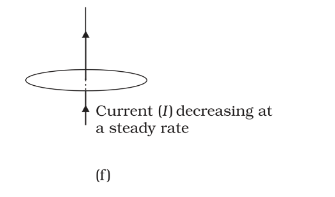Solution: The direction of induced emf (current) can be found from Lenz's law. According to this law, the direction of induced emf is as shown in the following figures, when the north pole of a magnet approaching and receding respectively.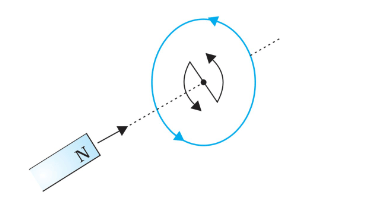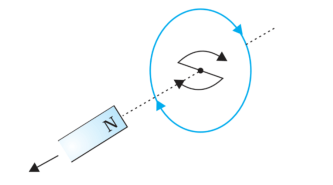Using Lenz's law, the diction of induced current in the different situations given is predicted as following.

(a) Along qrpq

(b) Along prqp

(c) Along xyzx

(d) Along xzyx

(e) Along xryx

(f) No current is induced as no field lines are crossing the loop.

Question 2. Use Lenz's law to determine the direction of induced current in the situations described by Fig. 6.19:

(a) A wire of irregular shape turning into a circular shape:

(b) A circular loop being deformed into a narrow straight wire.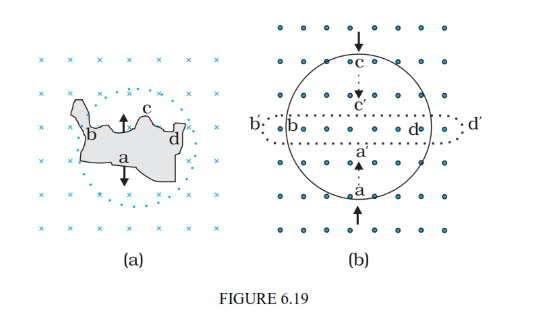Solution: (a) In this case, the area is increasing. Hence flux through the loop also increases during the shape change. According to Lenz's law induced current direction is in such a way that, it opposes the change in flux. Hence the induced current will be along adcba

(b) In this case, the area is decreasing. Hence flux through the loop also decreases during the shape change. According to Lenz's law induced current direction is in such a way that, it opposes the change in flux. Hence the induced current will be along $\mathrm{a}^{\prime} \mathrm{d}^{\prime} \mathrm{c}^{\prime} \mathrm{b}^{\prime} \mathrm{a}^{\prime}$

Question 3. A long solenoid with 15 turns per cm has a small loop of area $2.0 \mathrm{~cm}^{2}$ placed inside the solenoid normal to its axis. If the current carried by the solenoid changes steadily from $2.0 \mathrm{~A}$ to $4.0 \mathrm{~A}$ in $0.1 \mathrm{~s}$, what is the induced emf in the loop while the current is changing?

Solution: Given that,

Number of turns per unit length, $n=15$ per $\mathrm{cm}=1500$ per $\mathrm{m}$

Area of the loop $A=2.0 \mathrm{~cm}^{2}=2.0 \times 10^{-4} \mathrm{~m}^{2}$

Change in the current of the solenoid $\Delta i=4.0 \mathrm{~A}-2.0 \mathrm{~A}=2.0 \mathrm{~A}$

Time taken for this change $\Delta t=0.1 \mathrm{~s}$

emf induced in the small loop placed $\varepsilon$

$=\frac{\Delta \phi}{\Delta t}=A \times \frac{\Delta B}{\Delta t}=A \times \mu_{0} \times n \times \frac{\Delta i}{\Delta t}$

$\Rightarrow \varepsilon=2.0 \times 10^{-4} \times 4 \pi \times 10^{-7}$

$\times 1500 \times \frac{2.0}{0.1}$

$\Rightarrow \varepsilon=7.5 \times 10^{-6} \mathrm{~V}$

$\therefore$ emf induced in the small loop placed $\varepsilon=7.5 \times 10^{-6} \mathrm{~V}$

Question 4. A rectangular wire loop of sides $8 \mathrm{~cm}$ and $2 \mathrm{~cm}$ with a small cut is moving out of a region of uniform magnetic field of magnitude $0.3 \mathrm{~T}$ directed normal to the loop. What is the emf developed across the cut if the velocity of the loop is $1 \mathrm{~cm} \mathrm{~s}^{-1}$ in a direction normal to the (a) longer side, (b) shorter side of the loop? For how long does the induced voltage last in each case?

Solution:(a)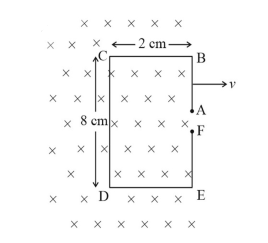When the loop is moving normal to the long side,

emf induced in the parts $\mathrm{CB}, \mathrm{DE}$ is zero as their lengths are parallel to the velocity, and also emf induced in $\mathrm{EB}$ is zero as it is out of the magnetic field.

Hence emf induced in the loop (across $\mathrm{AF})=$ emf induced across $\mathrm{CD}=\varepsilon=$ $v \times B \times l$

$\Rightarrow \varepsilon=1 \times 10^{-2} \times 0.3 \times 8 \times 10^{-2}$

$\Rightarrow \varepsilon=2.4 \times 10^{-4} \mathrm{~V}$

After 2 seconds, the side CD comes out of the magnetic field.

Hence the emf lasts for 2 seconds

(b)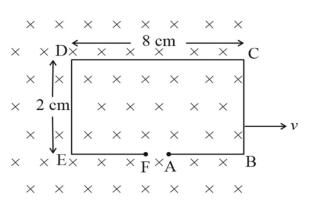When the loop is moving normal to the shorter side,

emf induced in the parts $\mathrm{EB}, \mathrm{CD}$ is zero as their lengths are parallel to the velocity, and also emf induced in $\mathrm{BC}$ is zero as it is out of the magnetic field.

Hence emf induced in the loop (across AF) = emf induced across $\mathrm{ED}=\varepsilon=$ $v \times B \times l$

$\Rightarrow \varepsilon=1 \times 10^{-2} \times 0.3 \times 2 \times 10^{-2}$

$\Rightarrow \varepsilon=0.6 \times 10^{-4} \mathrm{~V}$

After 8 seconds, the side DE comes out of the magnetic field.

Hence the emf lasts for 8 seconds

Question 5. A $1.0 \mathrm{~m}$ long metallic rod is rotated with an angular frequency of $400 \mathrm{rad} \mathrm{s}^{-1}$ about an axis normal to the rod passing through its one end. The other end of the rod is in contact with a circular metallic ring. A constant and uniform magnetic field of $0.5 \mathrm{~T}$ parallel to the axis exists everywhere. Calculate the emf developed between the centre and the ring.

Solution. Given that,

\text { Angular frequency } \omega=400 \mathrm{rad} \mathrm{s}^{-1}

Length of the metallic rod $l=1 \mathrm{~m}$

emf induced across the rotating rod,

$\varepsilon=\frac{1}{2} \omega B l^{2}$

$\varepsilon=\frac{1}{2} \times 400 \times 0.5 \times 1^{2}$

$\varepsilon=100 \mathrm{~V}$

$\therefore$ emf developed between the centre and the ring, $\varepsilon=100 \mathrm{~V}$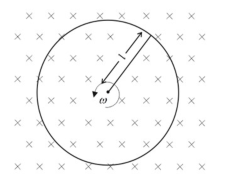Question 6. A circular coil of radius $8.0 \mathrm{~cm}$ and 20 turns is rotated about its vertical diameter with an angular speed of $50 \mathrm{rad} \mathrm{s}^{-1}$ in a uniform horizontal magnetic field of magnitude $3.0 \times 10^{-2} \mathrm{~T}$. Obtain the maximum and average emf induced in the coil. If the coil forms a closed loop of resistance $10 \Omega$, calculate the maximum value of current in the coil. Calculate the average power loss due to Joule heating. Where does this power come from?

Solution. Given,

The circular coil radius, $r=8 \mathrm{~cm}=0.08 \mathrm{~m}$

Area of the coil, $A=\pi r^{2}=\pi \times(0.08)^{2} \mathrm{~m}^{2}$

Number of turns on the coil, $N=20$

Angular speed, $\omega=50 \mathrm{rad} / \mathrm{s}$

Strength of magnetic, $B=3 \times 10^{-2} \mathrm{~T}$

Total resistance produced by the loop, $R=10 \Omega$

emf induced in the loop $\varepsilon=-N \omega A B \sin (\omega t)$

$\Rightarrow$ Maximum emf induced in the loop $\varepsilon_{\max }=N \omega A B=20 \times 50 \times \pi \times(0.08)^{2} \times$ $3 \times 10^{-2}$

$\Rightarrow \varepsilon_{\max }=0.603 \mathrm{~V}$

Over a full cycle, the average emf induced in the coil is zero.

The maximum current in the loop $I_{\max }=\frac{\varepsilon_{\max }}{R}=\frac{0.603 \mathrm{~V}}{10 \Omega}=0.0603 \mathrm{~A}$

Average power loss due to Joule heating, $P_{\text {avg }}=\frac{1}{2} \times \varepsilon_{\max } \times I_{\max }=\frac{1}{2} \times 0.603 \times$

$0.06032$

$\Rightarrow P_{\text {avg }}=0.018 \mathrm{~W}$

The current induced in the loop causes torque opposing the rotation of the coil. In order to rotate the loop continuously at a uniform rate, an external agent (rotor) must supply torque (and do work). Thus, the source of the power dissipated as heat in the coil is the external rotor.

Question 7. A horizontal straight wire $10 \mathrm{~m}$ long extending from east to west is falling with a speed of $5.0 \mathrm{~m} \mathrm{~s}^{-1}$, at right angles to the horizontal component of the earth's magnetic field, $0.30 \times 10^{-4} \mathrm{~Wb} \mathrm{~m}^{-2}$. (a) What is the instantaneous value of the emf induced in the wire? (b) What is the direction of the emf? (c) Which end of the wire is at the higher electrical potential?

Solution. Given,

Length of the wire, $l=10 \mathrm{~m}$

Speed of the wire with which it is falling, $v=5.0 \mathrm{~m} / \mathrm{s}$

Strength of magnetic field, $B=0.3 \times 10^{-4} \mathrm{~Wb} \mathrm{~m}^{-2}$

(a) emf induced in the wire $\varepsilon=v \times B \times l=5.0 \times 0.3 \times 10^{-4} \times 10$

$\Rightarrow \varepsilon=1.5 \times 10^{-3} \mathrm{~V}$

(b) The direction of the emf is from West to East.

(c) The end towards the east has higher potential.

Question 8. Current in a circuit falls from $5.0 \mathrm{~A}$ to $0.0 \mathrm{~A}$ in $0.1 \mathrm{~s}$. If an average emf of $200 \mathrm{~V}$ induced, give an estimate of the self-inductance of the circuit.

Solution. Given,

Average emf $\varepsilon_{\text {avg }}=200 \mathrm{~V}$

Change in current $\Delta I=5.0-0.0=5 \mathrm{~A}$

Time taken for the change in current $\Delta t=0.1 \mathrm{~s}$

Average emf induced across an inductor, $\varepsilon_{\text {avg }}=L \times \frac{\Delta l}{\Delta t}$

$\Rightarrow 200 \mathrm{~V}=L \times \frac{5 \mathrm{~A}}{0.1 \mathrm{~s}}$

$\Rightarrow L=4 \mathrm{H}$

$\therefore$ self-inductance of the circuit is $4 \mathrm{H}$

Question. 9. A pair of adjacent coils has a mutual inductance of $1.5 \mathrm{H}$. If the current in one coil changes from 0 to $20 \mathrm{~A}$ in $0.5 \mathrm{~s}$, what is the change of flux linkage with the other coil?

Solution. Given,

The mutual inductance $M=1.5 \mathrm{H}$

Change in current in the first coil, $\Delta l_{1}=20 \mathrm{~A}-0 \mathrm{~A}=20 \mathrm{~A}$

Time taken for the current change $\Delta t=0.5 \mathrm{~s}$

Change in flux linked with the second coil, $\Delta \phi_{2}=M \times \Delta I_{1}=1.5 \mathrm{H} \times 20 \mathrm{~A}$

$\Rightarrow \Delta \phi_{2}=30 \mathrm{~Wb}$

$\therefore$ Change of flux linked with the second coil is $30 \mathrm{~Wb}$

Question 10. A jet plane is travelling towards west at a speed of $1800 \mathrm{~km} / \mathrm{h}$. What is the voltage difference developed between the ends of the wing having a span of $25 \mathrm{~m}$, if the Earth's magnetic field at the location has a magnitude of $5 \times 10^{-4} \mathrm{~T}$ and the dip angle is $30^{\circ}$.

Solution. Given,

Speed of the plane, $v=1800 \mathrm{~km} / \mathrm{h}=1800 \times \frac{5}{18} \mathrm{~m} / \mathrm{s}=500 \mathrm{~m} / \mathrm{s}$

Length of the wingspan, $l=25 \mathrm{~m}$

Strength of the earth's magnetic field $B=5 \times 10^{-4} \mathrm{~T}$

Dip angle $\theta=30^{\circ}$

The vertical component of the magnetic field, $B_{\mathrm{V}}=B \sin 30^{\circ}=5 \times 10^{-4} \mathrm{~T} \times$

$\frac{1}{2}=2.5 \times 10^{-4} \mathrm{~T}$

emf induced across the wingspan, $\varepsilon=v \times B_{V} \times l=500 \mathrm{~m} / \mathrm{s} \times 2.5 \times 10^{-4} \mathrm{~T} \times$

$25 \mathrm{~m}=3.125 \mathrm{~V}$

$\therefore$ Voltage difference developed between the ends of the wingspan $=3.125 \mathrm{~V}$

NCERT Back of the Book Questions Additional Exercise:

Question 11. Suppose the loop in Exercise $6.4$ is stationary but the current feeding the electromagnet that produces the magnetic field is gradually reduced so that the field decreases from its initial value of $0.3 \mathrm{~T}$ at the rate of $0.02 \mathrm{~T} \mathrm{~s}^{-1}$. If the cut is joined and the loop has a resistance of $1.6 \Omega$, how much power is dissipated by the loop as heat? What is the source of this power?

Solution.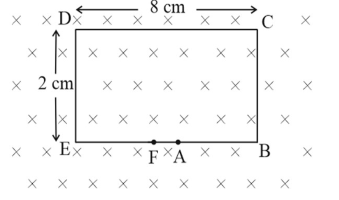Given,

The rate of change of the magnetic field $\frac{d B}{d t}=-0.02 \mathrm{~T} \mathrm{~s}^{-1}$

The resistance of the loop $R=1.6 \Omega$

Area of the loop $A=2 \mathrm{~cm} \times 8 \mathrm{~cm}=16 \times 10^{-4} \mathrm{~m}^{-2}$

Flux through the loop $\phi=A \times B$

The magnitude of the emf induced in the loop $|\varepsilon|=-\frac{d \phi}{d t}=A \times \frac{d B}{d t}=$ $16 \times 10^{-4} \mathrm{~m}^{-2} \times 0.02 \mathrm{~T} \mathrm{~s}^{-1}$

$=0.32 \times 10^{-4} \mathrm{~V}$

The power dissipated in the loop $P=\frac{|\varepsilon|^{2}}{R}=\frac{\left(0.32 \times 10^{-4}\right)^{2}}{1.6}=6.4 \times 10^{-10} \mathrm{~W}$

Source of this power is electromagnet, which is changing the magnetic field with time.

Question 12. A square loop of side $12 \mathrm{~cm}$ with its sides parallel to $\mathrm{X}$ and $\mathrm{Y}$ axes is moved with a velocity of $8 \mathrm{~cm} \mathrm{~s}^{-1}$ in the positive $\mathrm{x}$-direction in an environment containing a magnetic field in the positive z-direction. The field is neither uniform in space nor constant in time. It has a gradient of $10^{-3} \mathrm{~T} \mathrm{~cm}^{-1}$ along the negative $\mathrm{x}$-direction (that is it increases by $10^{-3} \mathrm{~T} \mathrm{~cm}^{-1}$ as one moves in the negative $\mathrm{x}$-direction), and it is decreasing in time at the rate of $10^{-3} \mathrm{~T} \mathrm{~s}^{-1}$. Determine the direction and magnitude of the induced current in the loop if its resistance is $4.50 \mathrm{~m} \Omega$.

Solution. Given,

Velocity of the loop, $v=8 \mathrm{~cm} \mathrm{~s}^{-1}=8 \times 10^{-2} \mathrm{~m} \mathrm{~s}^{-1}$

Area of the loop $A=12 \mathrm{~cm} \times 12 \mathrm{~cm}=144 \times 10^{-4} \mathrm{~m}^{2}$

emf in the loop is due to

(a) Change in the flux due to change in the magnitude of the magnetic field with time

(b) Change in the flux due to the magnetic field gradient along negative $\mathrm{x}-$ direction

Rate of change of flux due to variation in $B$ with time $=144 \times 10^{-4} \mathrm{~m}^{2} \times$ $10^{-3} \mathrm{~T} \mathrm{~s}^{-1}=1.44 \times 10^{-5} \mathrm{~Wb} \mathrm{~s}^{-1}$

From Lenz's law Induced emf will be in anticlockwise

Rate of change of flux due to the magnetic field gradient along negative $\mathrm{x}-$ direction

$=144 \times 10^{-4} \mathrm{~m}^{2} \times 8 \mathrm{~cm} \mathrm{~s}^{-1} \times 10^{-3} \mathrm{~T} \mathrm{~cm}^{-1}=$

$=11.52 \times 10^{-5} \mathrm{~Wb} \mathrm{~s}^{-1}$

From Lenz's law Induced emf will be in anticlockwise

Hence total emf induced $\varepsilon=1.44 \times 10^{-5}+11.52 \times 10^{-5}$

$=12.96 \times$

Induced current $I=\frac{\varepsilon}{R}=\frac{12.96 \times 10^{-5}}{4.50 \times 10^{-3}}$

$=2.88 \times 10^{-2} \mathrm{~A}$

The direction of the induced current is such that it should increase the flux along the positive z-direction. Hence for an observer watching the loop along the negative z-direction, the current appears to flow in the anticlockwise direction.

Question 13. It is desired to measure the magnitude of field between the poles of a powerful loud speaker magnet. A small flat search coil of area $2 \mathrm{~cm}^{2}$ with 25 closely wound turns, is positioned normal to the field direction, and then quickly snatched out of the field region. (Equivalently, one can give it a quick $90^{\circ}$ turn to bring its plane parallel to the field direction). The total charge flown in the coil (measured by a ballistic galvanometer connected to coil) is $7.5 \mathrm{mC}$. The combined resistance of the coil and the galvanometer is $0.50 \Omega$. Estimate the field strength of magnet.

Solution. Given,

Area of the $\operatorname{coil} A=2 \mathrm{~cm}^{2}=2 \times 10^{-4} \mathrm{~m}^{2}$

Number of turns of the coil $N=25$

Charge flown through the ballistic galvanometer $\Delta Q=7.5 \mathrm{mC}=7.5 \times 10^{-3} \mathrm{C}$

The resistance of the coil $R=0.50 \Omega$

Let's assume the magnitude of the magnetic field between the poles is $B$

Change in flux when the loop is quickly snatched out of the field $\Delta \phi=$ $25 \times B \times\left(2 \times 10^{-4}-0\right)$

Charge flown through the coil $\Delta Q=\frac{1}{R} \Delta \phi$

$\Rightarrow 7.5 \times 10^{-3} \mathrm{C}=\frac{1}{0.50 \Omega} \times 25$

$\times B \times\left(2 \times 10^{-4}-0\right)$

$\Rightarrow B=0.75 \mathrm{~T}$

$\therefore$ The field strength of the magnetic field $B=0.75 \mathrm{~T}$

Question 14. Figure $6.20$ shows a metal rod $\mathrm{PQ}$ resting on the smooth rails $\mathrm{AB}$ and positioned between the poles of a permanent magnet. The rails, the rod, and the magnetic field are in three mutual perpendicular directions. A galvanometer G connects the rails through a switch $K$. Length of the rod $=15 \mathrm{~cm}, B=0.50 \mathrm{~T}$, resistance of the closed loop containing the rod $=9.0 \mathrm{~m} \Omega$. Assume the field to be uniform.

(a) Suppose $\mathrm{K}$ is open, and the rod is moved with a speed of $12 \mathrm{~cm} \mathrm{~s}^{-1}$ in the direction shown. Give the polarity and magnitude of the induced emf.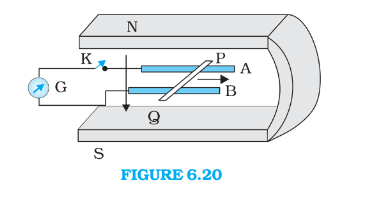(b) Is there an excess charge built up at the ends of the rods when $\mathrm{K}$ is open? What if $\mathrm{K}$ is closed?

(c) With $\mathrm{K}$ open and the rod moving uniformly, there is no net force on the electrons in the rod PQ even though they do experience magnetic force due to the motion of the rod. Explain.

(d) What is the retarding force on the rod when $\mathrm{K}$ is closed?

(e) How much power is required (by an external agent) to keep the rod moving at the same speed $\left(=12 \mathrm{~cm} \mathrm{~s}^{-1}\right)$ when $\mathrm{K}$ is closed? How much power is required when $\mathrm{K}$ is open?

(f) How much power is dissipated as heat in the closed circuit? What is the source of this power?

(g) What is the induced emf in the moving rod if the magnetic field is parallel to the rails instead of being perpendicular?

Solution. Given,

Length of the $\operatorname{rod} l=15 \mathrm{~cm}$

Magnetic field $B=0.50 \mathrm{~T}$

The resistance of the closed loop containing the $\operatorname{rod} R=9.0 \mathrm{~m} \Omega$

(a) $\quad$ Iduced emf $\varepsilon=v \times B \times l$

$=12 \times 10^{-2} \times 0.50 \times 15 \times 10^{-2}=90 \times 10^{-4} \mathrm{~V}$

$=9.0 \mathrm{mV}$

$P$ is the positive end, and $Q$ is the negative end.

(b) The excess charge is accumulated at the ends $(P$ and $Q$ ) when switch $K$ is open. When the switch is closed, excess charge is maintained by the continuous flow of the current.

(c) Electric field setup due to excess charge accumulated at the ends $(P$ and $Q)$ cancels the magnetic force.

(d) Current through the loop $I$

$=\frac{\varepsilon}{R}=\frac{9.0 \mathrm{mV}}{9.0 \mathrm{~m} \Omega}=1 \mathrm{~A}$

$\therefore$ The retarding force acting on the $\operatorname{rod} F=I \times l \times B$

$\Rightarrow F=1 \times 15 \times 10^{-2} \times 0.50=75 \times 10^{-3} \mathrm{~N}$

(e) The external force required = Magnetic force on the rod $=75 \times 10^{-3} \mathrm{~N}$

$\therefore$ Power required when the switch is closed $P=F \times v$

$\Rightarrow P=75 \times 10^{-3} \mathrm{~N} \times 12 \times 10^{-2} \mathrm{~m} \mathrm{~s}^{-1}=9.0 \times 10^{-3} \mathrm{~W}$

No power is required by an external agent when the switch is open.

(f) Power dissipated in the circuit $P=I^{2} R=1 \times 9.0 \times 10^{-3}=9.0 \times$ $10^{-3} \mathrm{~W}$

Source of this power is the external agent which is maintaining the constant speed

(g) If the magnetic field is parallel to the rails, speed of the rod is parallel to the magnetic field, which gives zero emf induced in the rod

Question 15. An air-cored solenoid with length $30 \mathrm{~cm}$, area of cross-section $25 \mathrm{~cm}^{2}$ and number of turns 500 , carries a current of $2.5 \mathrm{~A}$. The current is suddenly switched off in a brief time of $10^{-3} \mathrm{~s}$. How much is the average back emf induced across the ends of the open switch in the circuit? Ignore the variation in magnetic field near the ends of the solenoid.

Solution. Given,

The length of the solenoid $l=30 \mathrm{~cm}$

Area of cross-section $A=25 \mathrm{~cm}^{2}$

Number of turns $N=500$

Change in the current $\Delta I=2.5 \mathrm{~A}-0=2.5 \mathrm{~A}$

Time taken $\Delta t=10^{-3} \mathrm{~s}$

Back emf induced $|\varepsilon|=L \frac{\Delta I}{\Delta t}=\mu_{0}\left(\frac{N}{l}\right)^{2} A l \frac{\Delta I}{\Delta t}$

$=4 \pi \times 10^{-7} \times \frac{(500)^{2}}{30 \times 10^{-2}} \times 25 \times$

$10^{-4} \frac{2.5}{10^{-3}}$

$=6.5 \mathrm{~V}$

Question 16. (a) Obtain an expression for the mutual inductance between a long straight wire and a square loop of side a as shown in Fig. $6.21$.

(b) Now assume that the straight wire carries a current of $50 \mathrm{~A}$ and the loop is moved to the right with a constant velocity, $v=10 \mathrm{~m} / \mathrm{s} .$ Calculate the induced emf in the loop at the instant when $x=0.2 \mathrm{~m}$. Take $a=0.1 \mathrm{~m}$ and assume that the loop has a large resistance.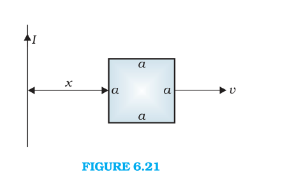Solution.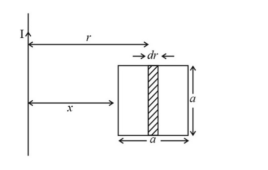Consider a small elemental area of width d $r$ between $r$ and $r+d r$

Magnetic field due to the current carrying long wire at a distance $r$ is $=\frac{\mu_{0} I}{2 \pi r}$

Flux through the elemental area, $\mathrm{d} \phi=\frac{\mu_{0} I}{2 \pi r} \times a \times \mathrm{d} r$

Total flux through the square loop, $\phi=\int_{x}^{x+a} \frac{\mu_{0} I}{2 \pi r} \times a \times \mathrm{d} r$

$\Rightarrow \phi=\frac{\mu_{0} I a}{2 \pi} \ln \left(\frac{x+a}{x}\right)$

$\therefore$ Mutual inductance $M=\frac{\phi}{I}=\frac{\mu_{0} a}{2 \pi} \ln \left(\frac{x+a}{x}\right)$

(b)

Given,

The wire is carrying a current $I=50 \mathrm{~A}$

Velocity of the loop $v=10 \mathrm{~m} / \mathrm{s}$

Induced emf $\varepsilon=\frac{\mu_{0} I}{2 \pi x} v a-\frac{\mu_{0} I}{2 \pi(x+a)} v a=\frac{\mu_{0} I}{2 \pi} v a\left(\frac{1}{x}-\frac{1}{x+a}\right)$

$\Rightarrow \varepsilon=2 \times 10^{-7} \times 50 \times 10 \times 0.1 \times\left(\frac{1}{0.2}-\frac{1}{0.2+0.1}\right)$

$\Rightarrow \varepsilon=1.7 \times 10^{-5} \mathrm{~V}$

Question 17. A line charge $\lambda$ per unit length is lodged uniformly onto the rim of a wheel of mass $\mathrm{M}$ and radius $\mathrm{R}$. The wheel has light non-conducting spokes and is free to rotate without friction about its axis (Fig. 6.22). A uniform magnetic field extends over a circular region within the rim. It is given by,

$B=-B_{0} \boldsymbol{k}$

(r is less then equal to a and a < R)

=0 (otherwise)

What is the angular velocity of the wheel after the field is suddenly switched off?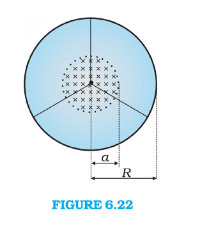Solution. Given charge per unit length $=\lambda$

Mass of the wheel $=M$

The radius of the wheel $=R$

From Faraday's law, induced electric filed along the rim when the filed is switched off

$\varepsilon=\oint \boldsymbol{E} . \mathrm{d} \boldsymbol{r}=-\frac{d \phi_{M}}{\overline{d t}}$

$\Rightarrow E \times 2 \pi R=-\pi a^{2} \frac{d B}{d t}$

$\Rightarrow E=-\frac{a^{2}}{2 R} \frac{d B}{d t}$

The torque acting on the wheel $\tau=E \times \lambda \times 2 \pi R \times R=2 \pi \lambda E R^{2}$

Angular velocity of the wheel $\omega=0+\left(\frac{2 \pi \lambda E R^{2}}{M R^{2}}\right) d t$

$\Rightarrow \omega=\frac{2 \pi \lambda R^{2}}{M R^{2}}\left(\frac{a^{2}}{2 R} \frac{d B}{d t}\right) d t$

$\Rightarrow \omega=-\frac{\pi \lambda a^{2} B_{0}}{M R} \boldsymbol{k}$